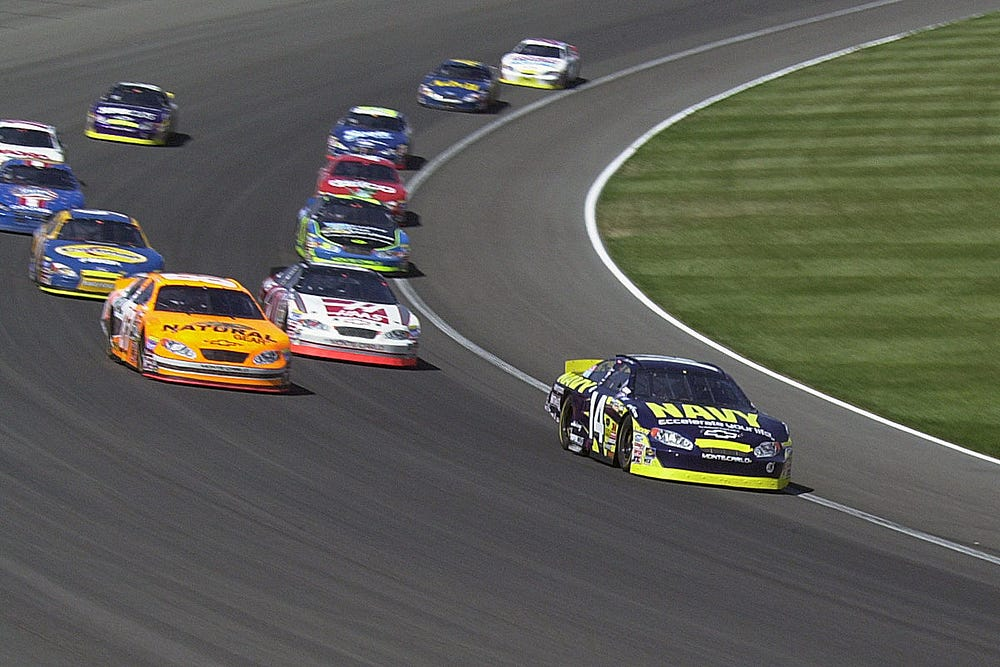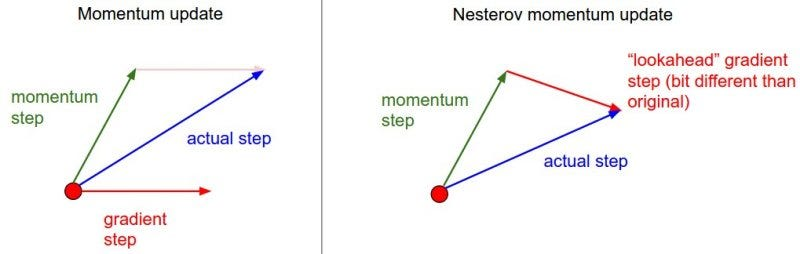[ML advanced]Momentum in machine learning? What is Nesterov momentum?

Source: Deep Learning on Medium

Momentum? As in the physics concept?

Wait, I signed up for machine learning, not this.

What

The basic idea of momentum in ML is to increase the speed of training.

This concept is one of those small bells and whistles that you think is not as important but turns out to be a real time saver and makes things go a lot smoother.

Why

It is mostly used in neural networks considering the size of data in NNs makes a more differentiable time difference while training gradients.

As the famous saying goes “Gotta go fast!”. Okay, in all seriousness, sometimes gradient descent can take ages when the dataset is sufficiently large.

Pros:

1. Can be used to handle noisy gradients
2. Can handle extremely small gradients

Cons:

1. Introduces further complexity in the model

How

Before we start, here is a small revision of gradient descent basics.

We will be discussing 2 types of updating techniques:

1. Simple momentum update
2. Nesterov momentum update

Before we get into the nitty-gritty, here is the vanilla gradient descent update:

``# Vanilla updatew += - learning_rate * dw``

Where,
`learning_rate` is a constant hyperparameter. The idea is to keep it low enough as to not overshoot the point of minima.

1. Simple momentum update

The physics class has started. Well, this is how it goes.

Think of the loss being a hilly roller coaster terrain, thus it has the potential energy of U.

U(potential energy) = mgh

Which simply implies that U(energy) ∝ h(height). We want that since when the gradient is on top, we want it to go to the bottom faster and when on the bottom of the curve, we want it to slow down in order to not miss the minima.

The force of the particle is considered as F = ma in the negative gradient

``# Momentum updatev = mu * v - learning_rate * dw # integrate velocityw += v # integrate position``

Where,
`v` is the particle that is initialized at zero. (from the top of the hill).
`mu` is referred to as momentum. Think of this as the coefficient of friction which will counteract v when it goes towards the bottom. Usually, the value is between (0.1–0.9). (Typically taken as 0.9).
This variable damps the energy of the system allowing v to stop.

Sometimes, we change the value of mu from 0.5 to 0.9 during multiple epochs to further optimize. it provides a relatively small boost to the speed of the system.

2. Nesterov momentum

This is a distant cousin of normal momentum update but it is quite popular owing to its consistency in getting the minima and the speed at which it does so.Car going 60km/hr in a straight line will end up 60 km from the origin in an hour

So, the core concept of Nesterov momentum lies in the fact that if you know the velocity and direction of an object, you can predict its location in time T.(left)The old way. Instead of going towards the gradient step, sometimes the movement is towards a different direction thus wasting time (right) Nesterov momentum calculates the step to be taken in future and takes the corrective action

Say the current vector at position x, velocity is `mu * v` . To predict where this point will end up in time t(the next step basically) will be `x + mu * v` . We can use this as a ‘lookahead’ or a future prediction for the point. Thus, we can adjust the movement of the gradient accordingly to get us to the right position.

``w_ahead = w+ mu * v# evaluate dx_ahead (the gradient at w_ahead instead of at w)v = mu * v - learning_rate * dw_aheadw += v` `

Well, you made it to the end. Nice! Thanks for the read.

Any suggestions for a more streamlined process or any doubts, please feel free to comment.